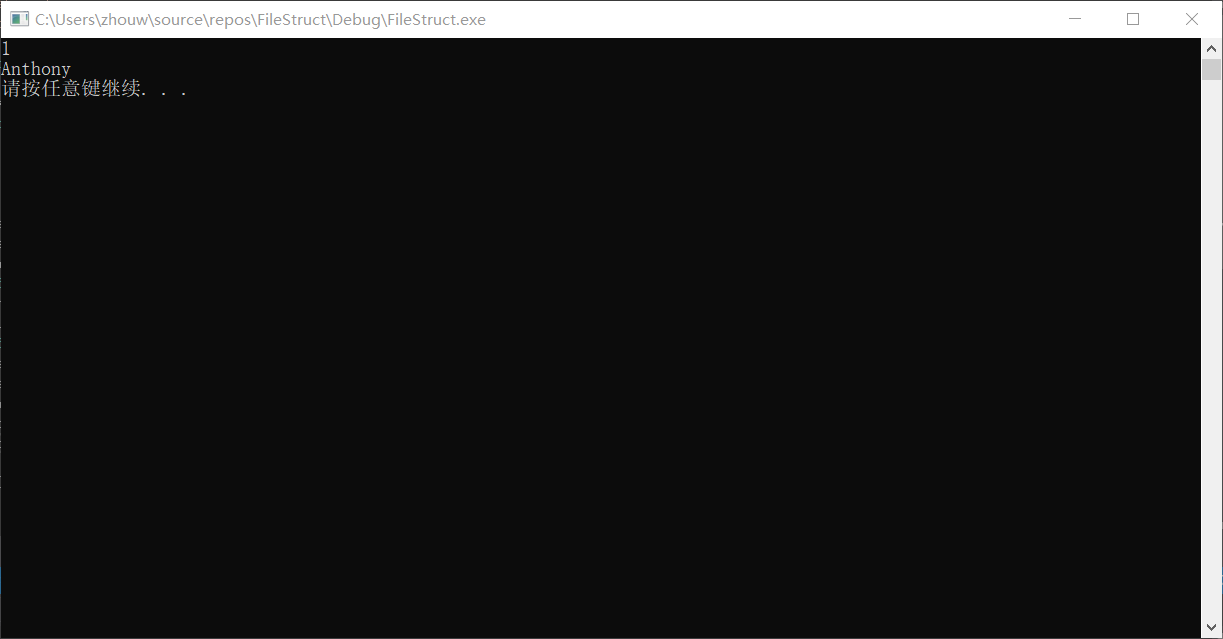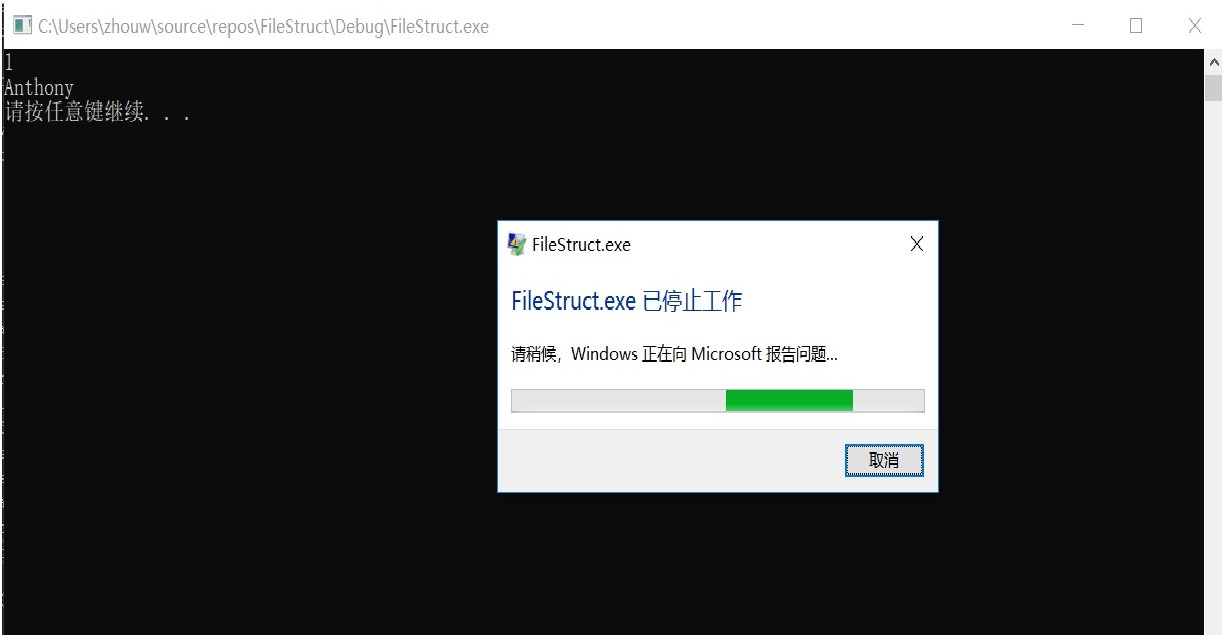C++中使用二进制文件保存和读取结构体的问题

#include
#include
#include

``````struct Test
{
int key;
std::string data;
};

int main()
{
Test test;
test.key = 1;
test.data = "Anthony";
std::ofstream fout("D:\\Data.dat", std::ios_base::out | std::ios_base::binary);
fout.write((char*)&test, sizeof Test);
fout.close();
std::ifstream fin("D:\\Data.dat", std::ios_base::in | std::ios_base::app | std::ios_base::binary);
Test test_1;
fin.seekg(0);
std::cout << test_1.key << std::endl;
std::cout << test_1.key << std::endl;
std::cout << test_1.data << std::endl;
std::cout << test_1.data << std::endl;

system("pause");
return 0;
}
``````3个回答

test.data = "Anthony";

string s = "Anthony";
test.data = s;-

-